# Common Core HS Algebra: Linear Equations Chapter Exam

Exam Instructions:

Choose your answers to the questions and click 'Next' to see the next set of questions. You can skip questions if you would like and come back to them later with the yellow "Go To First Skipped Question" button. When you have completed the practice exam, a green submit button will appear. Click it to see your results. Good luck!

### Page 1

#### Question 2 2. What is the equation of the graph below?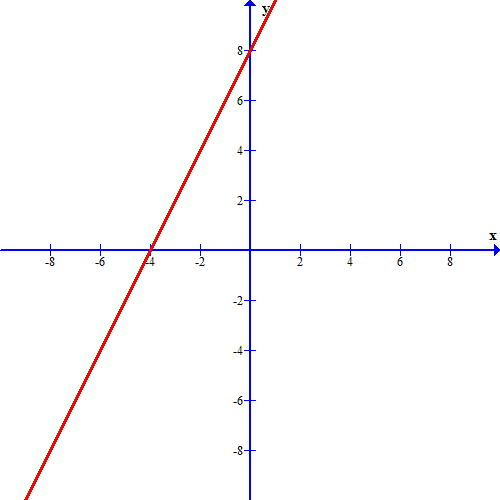#### Question 5 5. What is the slope-intercept form equation of the graph below?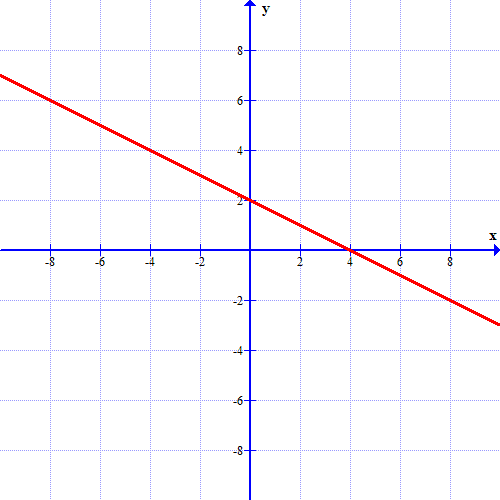### Page 4

#### Question 16 16. If the graph below has the equation Ax + By = -4, what must be true about A and B?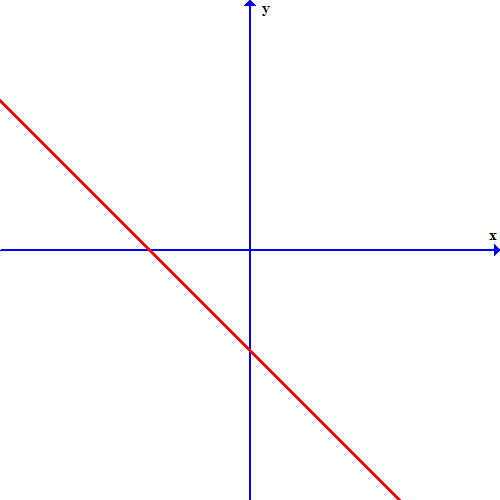#### Question 20 20. What are the coordinates of this point?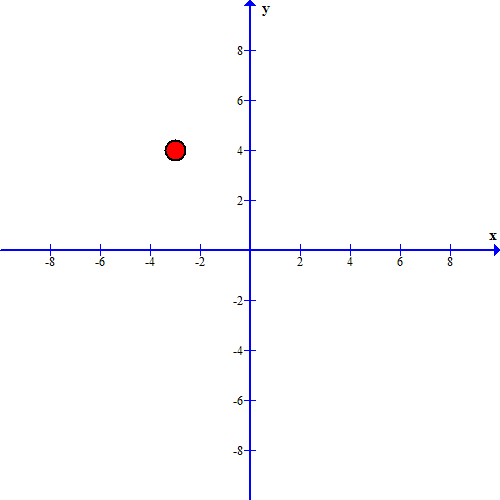### Page 6

#### Question 30 30. Which of these equations represents a line that is perpendicular to the line in the graph below?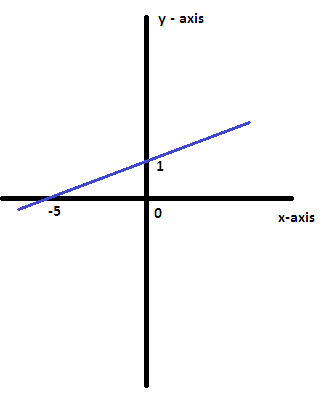#### Common Core HS Algebra: Linear Equations Chapter Exam Instructions

Choose your answers to the questions and click 'Next' to see the next set of questions. You can skip questions if you would like and come back to them later with the yellow "Go To First Skipped Question" button. When you have completed the practice exam, a green submit button will appear. Click it to see your results. Good luck!

Support